## Most Used Formulas (cont.)

 Coupled Inductance (cont.) Resonance (cont.) where      L = the total inductance,                  M = the mutual inductance,      L1 and L2 = the self inductance of                                                        the individual coils. where      F = the resonant frequency                         in cycles per second,                   L = inductance in henrys,                  C = capacitance in farads,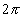= 6.28,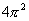= 39.5, Mutual Inductance Reactance The mutual inductance of two r-f coils with fields  interacting, is given by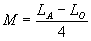where    M = mutual inductance, expressed                       in same units as LA and LO,              LA = Total inductance of coils                       L1 and L2 with fields aiding,              LO = Total inductance of coils                       L1 and L2 with fields opposing, of an inductance is expressed by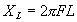of an capacitance is expressed by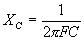where   XL =  inductive reactance in ohms,                         (known as positive reactance),               XC =  capacitive reactance in ohms,                         (known as negative reactance),                F  =  frequency in cycles per second,                L  =  inductance in henrys,                C  =  capacitance in farads,=  6.28, Coupling Coefficient Frequency from Wavelength When two r-f coils are inductively coupled so as to  give transformer action, the coupling coefficient is expressed by,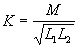where    K  =  the coupling coefficient;                         (K x 102 = coupling                         coefficient in %),                M  =  the mutual inductance value,    L1 and L2  =  the self-inductance of the two coils                         respectively, both being expressed                         in the same units.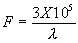where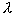=  wavelength in meters.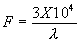where=  wavelength in centimeters. Resonance Wavelength from Frequency The resonant frequency, or frequency at which  inductive reactance XL equals capacitive reactance  XC is expressed by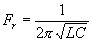also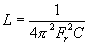and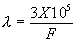where    F =  frequency in kilocycles.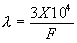where    F =  frequency in megacycles.